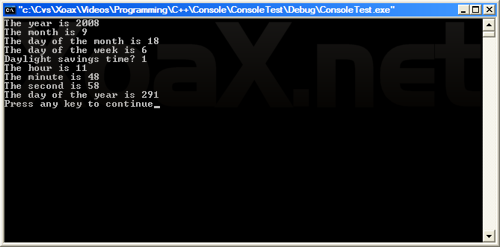• Programming
• Internet

# localtime()

### Declaration

`tm* localtime(const time_t* kqpTime);`

### Description

This function converts a time_t argument into a tm data type, which represents the local time. The passed in argument, kqpTime, is a pointer to a time_t variable which specifies number of seconds elapsed since midnight January 1, 1970. The time_t is value is calculated with the time() function. The value returned is a pointer to a tm struct variable that specifies the time with the seconds broken down into seconds, minutes, hours, etc. according to the tm struct definition.

### Example

```#include <iostream>
#include <ctime>

int main() {
using namespace std;

time_t qTime;
tm* qpTimeSpec;
// Get the number of seconds since midnight Jan. 1, 1970
time(&qTime);
// Convert the seconds to a tm struct variable
qpTimeSpec = localtime(&qTime);
cout << "The year is " << (1900 + qpTimeSpec->tm_year) << endl;
cout << "The month is " << qpTimeSpec->tm_mon << endl;
cout << "The day of the month is " << qpTimeSpec->tm_mday << endl;
cout << "The day of the week is " << qpTimeSpec->tm_wday << endl;
cout << "Daylight savings time? " << qpTimeSpec->tm_isdst << endl;
cout << "The hour is " << qpTimeSpec->tm_hour << endl;
cout << "The minute is " << qpTimeSpec->tm_min << endl;
cout << "The second is " << qpTimeSpec->tm_sec << endl;
cout << "The day of the year is " << qpTimeSpec->tm_yday << endl;

return 0;
}```

### Output